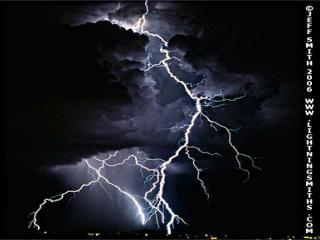DownloadDownload PresentationCurrent

# Current

Télécharger la présentation## Current

- - - - - - - - - - - - - - - - - - - - - - - - - - - E N D - - - - - - - - - - - - - - - - - - - - - - - - - - -
##### Presentation Transcript

1. Current

2. Current • There are two kinds of current:

3. Current • There are two kinds of current: • Direct current

4. Current • There are two kinds of current: • Direct current • Alternating current

5. Direct current

6. Direct current • DC flows in one direction • DC is the kind of current that comes from batteries.

7. Alternating current

8. Alternating current • AC flows back and forth rapidly. • In North America it flow back and forth 60 times a second. This is called 60 Hertz

9. Why do we use AC instead of DC?

10. Why do we use AC instead of DC?

11. Why do we use AC instead of DC?

12. Why do we use AC instead of DC?

13. Why do we use AC instead of DC?

14. Why we use AC instead of DC • AC can be more easily transmitted over long distances than DC.

15. Why we use AC instead of DC • AC can be more easily transmitted over long distances than DC. • Why?

16. Why we use AC instead of DC • AC can be more easily transmitted over long distances than DC. • Why? • Because of transformers

17. Transformers

18. Step-up transformers • Turns lower voltages into higher ones • Fewer turns on the primary side than the secondary one.

19. Step-up transformers

20. Step-down transformers • Turns higher voltages into lower ones. • More turns on the primary side than the secondary one.

21. Step-down transformers

22. Step-down transformers

23. Electromagnetic Induction

24. Electromagnetic Induction • E.i. describes the production of electricity by using a magnetic field. • This is how generators “make” electricity. • Generators convert mechanical energy into electrical energy.

25. Electromagnetic Induction

26. Electromagnetic Induction

27. POWER

28. POWER • Power is the rate at which devices use energy.

29. POWER • Power is the rate at which devices use energy. • The unit of power measurement is the watt (W). It measures how many units of energy are used per second.

30. POWER • Power is the rate at which devices use energy. • The unit of power measurement is the watt (W). It measures how many units of energy are used per second. • Energy is measured in joules (J)

31. POWER • The power rating of a device is directly proportional to the current flowing through the device and the voltage in the circuit.

32. POWER • The power rating of a device is directly proportional to the current flowing through the device and the voltage in the circuit. • In other words...

33. POWER • The power rating of a device is directly proportional to the current flowing through the device and the voltage in the circuit. • In other words... The Power Formula Power = current x voltage P = I x V

34. Math Time!!

35. Now, stop writing (and complaining) and watch this.